Fuzzy Support Vector Machine     Last updated on 2017/2560112, a full moon day;

1st to understand 4 sufficiency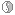4 deficiency conditions in plane of existence [Buddha\Book\Abhidhamma in Daily Life; 7.2.12, Pg. 71, Chapter 7]; Higher Planes & Lower Planes, also see: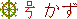; 4 planets prediction; Existence of hyper plane and hyperspace [also see: Orbit];

2nd to understand 24 mm natural time in no moon day and full moon day; also see: time;

3rd to understand computing {set} and computing fuzzy set {...... [Also see: distribution for simulation]

Therefore,

Fuzzy set { ... for hyperspace1 : WATER , Human_Beings_Line

Fuzzy set { ... for hyperspace2 : NO WATER , The_Sun_Line

Fuzzy set { ... for hyperspace3 : NO WATER , Humanoid_Line

4th to understand a Myanmar's imaginary dimensional hyperspace craft becomes in vector ... ;

5th to understand the book's mathematical classification i.e.

4 planets are classified into hyper_plane_1, and hyper_plane_2 Also read: Prof. Kaku's Parallel World

The 1st crack of our universe as a tangent line therefore named as The_Sun_Line, and notice that the gap [very thin layer to survive as human beings] therefore named as Human_Beings_Line, and the space line therefore named as Humanoid_Line;

At this present in ACT2 stage, 3 lines are The_Sun_Line, Human_Beings_Line, and Humanoid_Line;

At this present in ACT2 stage, 2 hyper planes are hyper_plane_1, and hyper_plane_2, and each hyper_plane has yin-yang Shinto effect of 2 planets synchronizing each other for very very long period of time already ... ;

According to Buddha's dhamma, a middle path way should be kept, therefore the following image [a combination of hyper plane 1 AND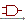2 ] is in neither no moon day nor full moon day;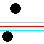hyperplane2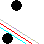For hyperplane1, WHERE The_Sun_Line, just before noisy dB, as a daily routine crack to ice, the 1st crack WHICH breaks symmetries, ... along with Human_Beings_Line, water, ... along with Humanoid_Line in space; For each hyper plane, also see: pairs of sticks ... ;

Human_Beings_Line : Kf(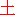) + b = 1

The_Sun_Line : Kf() + b = 0

Humanoid_Line  : Kf() + b = -1

K kernel mapping function f prompts fuzzy parameters K and b to determine position of 4 planets prediction;

Linear data as: Δi0 is equal to (i1 -01, ... ,in -0n)  determines

f(Δi0) is equal to (f1i0), ... , fNi0))

Distance between lines are 1/||K||

1/||K|| is equal to ( i=1 Σ N ik2)1/2 for parameterized K values of K is equal to (k1, k2, k3, ... , kN)

||K||min value satisfies quadratic programming i.e.

Min <K, K>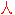i ( < Kf(i) > + b ) bigger than or equal to 1, WHERE i  is equal to 1, 2, 3, ... , counter's constraint integer number, therefore inner product is <.,.> ANDi corresponds to linear_entity_labeli0

For a Myanmar's imaginary dimensional hyperspace craft, its physical kinetic potential can be constructed by Lagrangian function to find K and b, therefore

Lagrangian (K, b, ACT1) is equal to 1/2 < K , K> - i=1Σyin-yang ACT1i [i ( < K, f(i) > + b ) - 1] WHERE ACT1i is bigger than or equal to zero

Therefore saddle point condition by Kuhn-Tucker satisfies

Lagrangian (K*, b*, ACT1) less than or equal to Lagrangian (K*, b*, ACT1*) less than or equal to Lagrangian (K, b, ACT1*)

Optional solution h* is equal to (K*, b*), therefore a middle path (K*, b*, ACT1*) by dhamma prompts that

MIN value of Lagrangian respects to K and b ANDMAX value of Lagrangian respects to ACT1

IFF d Lagrangian / d K is equal to d Lagrangian / d b is equal to zero, and then

K* is equal to i=1Σcounter's constraint integer number ACT1i*i f(i) ANDi=1Σcounter's constraint integer number ACT1i*i is equal to zero

By substituting, by solving quadratic programming,

values of ACT1* can be return {TRUE}

By using support vectors, by Karush-Kuhn-Tucker, b* vectors in hyperplanes can be solved

Therefore, inner product optimized form i.e.

i=1Σcounter's constraint integer number ACT1i*i < f(i) , f() > + b* is equal to zero

Therefore, IFF kernel function (i ,j) is equal to < f(i) , f() > Also read: Prof. Kaku's Parallel World

kernel function optimized form i.e.

i=1Σcounter's constraint integer number ACT1i*i kernel function (i ,j) + b* is equal to zero

Therefore, classifier general form i.e.

Class() is equal to sgn(i=1Σcounter's constraint integer number ACT1i*i kernel function (i ,j) + b* )

WHERE in ACT2 stage other 3 gravitational planets, before parallel time, before 3-in-1 dimensional hyperspace synchronization, before time risks in very very far away out of our universe, ...

 sgn () = { 1> zero 0is equal to zero -1< zero

Also read: 4.4.4 SVM; pg. 154 ~ 157; Foundations of Soft Case-Based Reasoning; Sankar Pal & Simon Shiu; Wiley Series on Intelligent Systems; 2004;

After 4 planets prediction, and ACT1 ACT2 stages, assuming dimensional hyperspace craft has gone very very very far away from our universe [4 planets prediction], and 5 sections horizontal gray scaled images prompts that in ACT 3 stage, further 2, 1, 0, -1, -2 kernel function can be developed for Fuzzy SVM ... , according to Buddha Dhamma, there will be uncountable universes ... ., therefore do good khamma, good results will ... .

For Monbusho level ACT2 stage dimensional hyperspace simulation developers only: develop higher fuzziness level such as 2, 1, 0, -1, -2 kernel function for each Lagrangian point by using fuzzy set { ... with distribution ANDgravitational machine's mass equilibrium.

Develop FuzzySet [2,2] [3,3,3] ... ; Also see: Fuzzy; Fuzzy [2,2] [3,3,3];

IFF laser gun, also see: hyperplane1's triangulate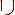distance;

...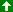Up